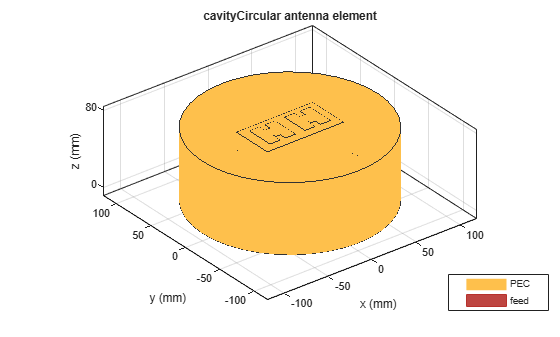# cavityCircular

Create circular cavity-backed antenna

## Description

Use the `circularCavity` object to create a circular cavity-backed antenna. By default, the exciter used is a dipole. The dimensions are chosen for an operating frequency of 1 GHz..

## Creation

### Syntax

``circularcavity = cavityCircular``
``circularcavity = cavityCircular(Name,Value)``

### Description

example

````circularcavity = cavityCircular` creates a circular cavity-backed antenna. ```

example

````circularcavity = cavityCircular(Name,Value)` sets properties using one or more name-value pairs. For example, `circularcavity = cavityCircular('Radius',0.2)` creates a circular cavity of radius 0.2 m. Enclose each property name in quotes.```

## Properties

expand all

Antenna type used as an exciter, specified as any single-element antenna object. Except reflector and cavity antenna elements, you can use any of the antenna elements or array elements in the Antenna Toolbox™ as an exciter.

Example: `'Exciter',horn`

Example: `ant.Exciter = horn`

Example: ```ant.Exciter = linearArray('patchMicrostrip')```

Radius of cavity, specified as a scalar in meters.

Example: `'Radius',0.2`

Example: `circularcavity.Radius = 0.2`

Data Types: `double`

Cavity height along z-axis, specified as a scalar in meters.

Example: `'Height',0.001`

Example: `circularcavity.Height = 0.001`

Data Types: `double`

Distance between the exciter and the base of the cavity, specified a scalar in meters.

Example: `'Spacing',7.5e-2`

Example: `circularcavity.Spacing = 7.5e-2`

Data Types: `double`

Type of dielectric material used as a substrate, specified as a object. For more information see, `dielectric`. For more information on dielectric substrate meshing, see Meshing.

Note

The substrate dimensions must be equal to the ground plane dimensions.

Example: ```d = dielectric('FR4'); 'Substrate',d```

Example: ```d = dielectric('FR4'); circularcavity.Substrate = d```

Create probe feed from backing structure to exciter, specified as `0` or `1` or a positive scalar. By default, probe feed is not enabled.

Example: `'EnableProbeFeed',1`

Example: ```circularcavity.EnableProbeFeed = 1```

Data Types: `double` | `logical`

Type of the metal used as a conductor, specified as a metal material object. You can choose any metal from the `MetalCatalog` or specify a metal of your choice. For more information, see `metal`. For more information on metal conductor meshing, see Meshing.

Example: ```m = metal('Copper'); 'Conductor',m```

Example: ```m = metal('Copper'); ant.Conductor = m```

Lumped elements added to the antenna feed, specified as a lumped element object. For more information, see `lumpedElement`.

Example: `'Load',lumpedelement`. `lumpedelement` is the object for the load created using `lumpedElement`.

Example: ```circularcavity.Load = lumpedElement('Impedance',75)```

Tilt angle of the antenna, specified as a scalar or vector with each element unit in degrees. For more information, see Rotate Antennas and Arrays.

Example: `'Tilt',90`

Example: `ant.Tilt = 90`

Example: `'Tilt',[90 90]`,`'TiltAxis',[0 1 0;0 1 1]` tilts the antenna at 90 degrees about the two axes defined by the vectors.

Note

The `wireStack` antenna object only accepts the dot method to change its properties.

Data Types: `double`

Tilt axis of the antenna, specified as:

• Three-element vector of Cartesian coordinates in meters. In this case, each coordinate in the vector starts at the origin and lies along the specified points on the X-, Y-, and Z-axes.

• Two points in space, each specified as three-element vectors of Cartesian coordinates. In this case, the antenna rotates around the line joining the two points in space.

• A string input describing simple rotations around one of the principal axes, 'X', 'Y', or 'Z'.

Example: `'TiltAxis',[0 1 0]`

Example: `'TiltAxis',[0 0 0;0 1 0]`

Example: `ant.TiltAxis = 'Z'`

Note

The `wireStack` antenna object only accepts the dot method to change its properties.

Data Types: `double`

## Object Functions

 `show` Display antenna or array structure; display shape as filled patch `axialRatio` Axial ratio of antenna `beamwidth` Beamwidth of antenna `charge` Charge distribution on metal or dielectric antenna or array surface `current` Current distribution on metal or dielectric antenna or array surface `design` Design prototype antenna or arrays for resonance around specified frequency `efficiency` Radiation efficiency of antenna `EHfields` Electric and magnetic fields of antennas; Embedded electric and magnetic fields of antenna element in arrays `impedance` Input impedance of antenna; scan impedance of array `mesh` Mesh properties of metal or dielectric antenna or array structure `meshconfig` Change mesh mode of antenna structure `optimize` Optimize antenna or array using SADEA optimizer `pattern` Radiation pattern and phase of antenna or array; Embedded pattern of antenna element in array `patternAzimuth` Azimuth pattern of antenna or array `patternElevation` Elevation pattern of antenna or array `rcs` Calculate and plot radar cross section (RCS) of platform, antenna, or array `returnLoss` Return loss of antenna; scan return loss of array `sparameters` Calculate S-parameter for antenna and antenna array objects `vswr` Voltage standing wave ratio of antenna

## Examples

collapse all

Create and view a default circular cavity-backed antenna.

`a = cavityCircular`
```a = cavityCircular with properties: Exciter: [1x1 dipole] Substrate: [1x1 dielectric] Radius: 0.1000 Height: 0.0750 Spacing: 0.0750 EnableProbeFeed: 0 Conductor: [1x1 metal] Tilt: 0 TiltAxis: [1 0 0] Load: [1x1 lumpedElement] ```
`show(a)`Create and view an equiangular spiral backed by a circular cavity. The cavity dimensions are:

Height = 0.01 m

Spacing = 0.01 m

``` ant = cavityCircular('Exciter',spiralEquiangular,'Radius',0.02, ... 'Height',0.01,'Spacing', 0.01); show(ant) ```Create a linear array of H-shaped patch microstrip antenna.

`arr = linearArray('Element',patchMicrostripHnotch,'ElementSpacing',0.04);`

Create a circular cavity-backed antenna with linear array exciter.

`ant = cavityCircular('Exciter',arr)`
```ant = cavityCircular with properties: Exciter: [1x1 linearArray] Substrate: [1x1 dielectric] Radius: 0.1000 Height: 0.0750 Spacing: 0.0750 EnableProbeFeed: 0 Conductor: [1x1 metal] Tilt: 0 TiltAxis: [1 0 0] Load: [1x1 lumpedElement] ```
`show(ant)`Create and visualize a circular cavity-backed cylindrical dielectric resonator antenna.

```e = draCylindrical; ant = cavityCircular('Exciter',e)```
```ant = cavityCircular with properties: Exciter: [1x1 draCylindrical] Substrate: [1x1 dielectric] Radius: 0.1000 Height: 0.0750 Spacing: 0.0750 EnableProbeFeed: 0 Conductor: [1x1 metal] Tilt: 0 TiltAxis: [1 0 0] Load: [1x1 lumpedElement] ```
`show(ant)`